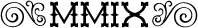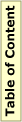## MMIX Bug Report UNSAVEmmix-20110831

### Bug Reported

Initial: 4/9/2013

Martin Ruckert

### Description

The UNSAVE instruction can enter an infinite loop.

### Details

The UNSAVE instruction loads the machine status from the register stack. The location of the register stack in memory is given by a global register. The first octa in memory determines the value of registers rA and rG. Both registers have restrictions on the possible values. The UNSAVE instruction as implemented in mmix-sim.w load new values from memory and does not check these limitations. (The implementation of UNSAVE in mmix-pipe.w does check the limitation, setting the B_BIT in rQ if the restrictions are violated.)

Specificaly, rG must not be less than 32. In the special case of loading zero into rG, the UNSAVE instruction enters an infinite loop in section 104, because rG (or G) is less or equal to rB.

``` for (k=rZ+1;;) {
@<Load |g[k]| from the register stack@>;
if (k==rP) k=rR;
else if (k==rB) k=255;
else if (k==G) break;
else k--;
}
```

### Proposed Patch

The code in mmix-sim.w should follow the code in mmix-pipe.w. This can be achived by the following patch to section 105:
```@x
if (k==rZ+1) x.l=G=g[rG].l=ll->tet>>24, a.l=g[rA].l=(ll+1)->tet&0x3ffff;
@y
if (k==rZ+1) {
x.l=G=g[rG].l=ll->tet>>24, a.l=g[rA].l=(ll+1)->tet&0x3ffff;
if (G<32)  x.l=G=g[rG].l=32;
}
@z
```
This will also prevent the infinite loop in the UNSAVE instruction.

### Discussion

The patch as given above is now part of the current sources.

Please help to keep this site up to date! If you want to point out important material or projects that are not listed here, if you find errors or want to suggest improvements, please send email to